# Rest and Motion

The general study of the relationship between motion, force and energy is known as mechanics. It is subdivided into three parts i.e. kinematics, dynamics and statics. Kinematics deals with the study of the motion of the objects without taking into account the forces and energies that may be involved. The first thing we need to know is that there is no absolute rest or absolute motion. Rest and motion are relative terms.

In simple terms, an object that changes its position is said to be in motion while the opposite action causes an object to be at rest. However, perspective plays a huge role in it. Take the example of an atom which is always in motion but when we see an object in the macro world it seems to be at rest. Therefore, rest and motion both depend mainly on the frame of reference of the one who is observing the scene.

## Rest and Motion Definitions

Rest: An object is said to be at rest if it does not change its position with respect to its surroundings with time.

Motion: An object is said to be in motion if the position changes with respect to it surrounding and time.

## Frame of Reference

Suppose an object is placed on the table, it can be said that the object is at rest. If a person is standing on the moon he will find that earth is changing its position continuously and so are the table and the object. Thus, to locate the position of anything one needs to define a frame of reference. For instance, if we take the earth as a reference, then the object is at rest but if the moon is taken as reference, the object is in motion. A frame of reference can be inertial or non-inertial depending on whether it is at rest or constantly moving.

## Rest and Motion Related Terms

### Speed

The distance covered by a moving body in a unit time interval is called its speed. It can be either uniform or non-uniform. Its units are meters per second (m/s)

Speed = [Distance Travelled]/[Time Taken]

Speed is generally shown in a position-time graph.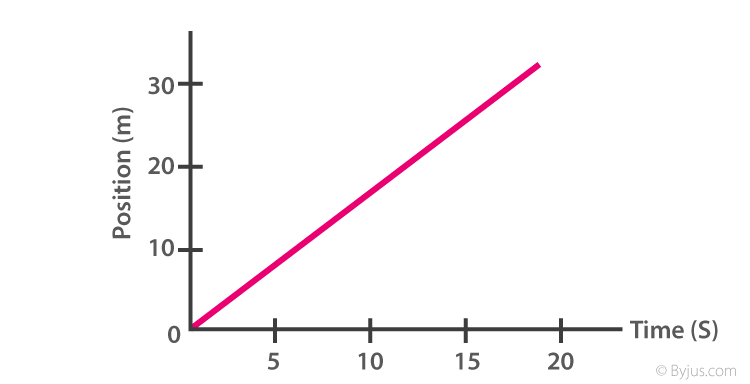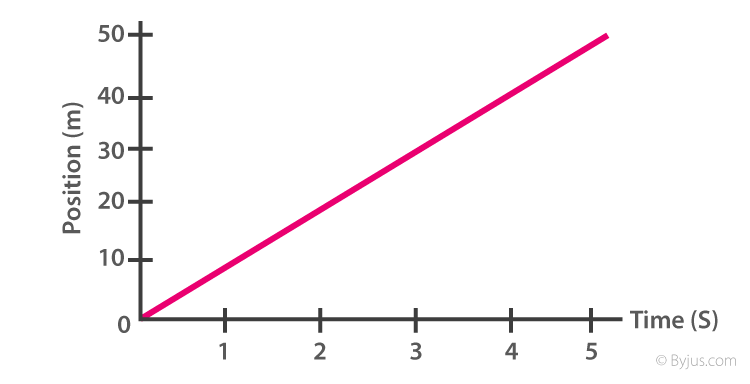• Uniform motion: When a body covers equal distance in an equal interval of time.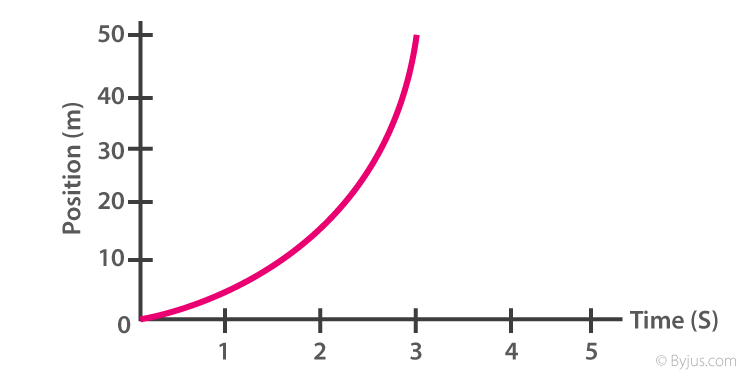• Non-Uniform Motion: Body covers an unequal distance in equal interval of time.

### Displacement

It is defined as the shortest distance between the initial position and the final position.

### Velocity

Change in the displacement of the body with respect to time is called its velocity. it is a vector quantity i.e. it has both magnitude and direction.

Velocity = Displacement / Time

• Uniform velocity: Magnitude and direction remain the same with respect to time.
• Non-Uniform velocity: When the body covers unequal displacement in an equal interval of time in a particular direction or if the direction changes, it is said to be moving at non-uniform velocity.
• Average velocity = Time displacement / Total Time Taken

### Acceleration and Deceleration

A body is said to be accelerating if it gradually increases its velocity with respect to time.

Average Acceleration = [Change in Velocity]/[Time taken] = Δy/Δt

Instantaneous acceleration = $a =\lim_{\Delta t \rightarrow 0}\frac{\Delta v}{\Delta t}$

### Centripetal Acceleration

When a body moves along a circular path of the radius R with constant speed (v), the velocity of the body keeps changing since the direction of the motion keeps changing. This change in velocity causes the body to experience an acceleration which is directed towards the centre of the circular path. This acceleration is called centripetal acceleration.

## Equations of Uniformly Accelerated Motion

(Along straight line) If a body started its motion with initial velocity ‘u’ and attains final velocity ‘v’ in the time interval ‘t’. The assumption used in all the equations is that the body covers distance ‘s’ at constant acceleration.

These equations are as follows:

• V = u + at
• S = ut + ½ at2
• V2 = u2 + 2as

Important points:

• Acceleration and deceleration are vector quantities with SI unit m / s2.
• A body is said to be decelerating if its velocity decreases with time.
• If acceleration does not change with time, it is said to be constant acceleration.
• Replace ‘a’ with acceleration due to gravity ‘g’ for a freely falling body. Similarly, if the body is thrown vertically upwards replace ‘a’ with ‘-g’.
• The slope of the displacement-time graph gives velocity and slope of the velocity-time graph give acceleration.

## Displacement, Velocity and Time Graphs

Different cases of the displacement-time graph (is the angle of the slope).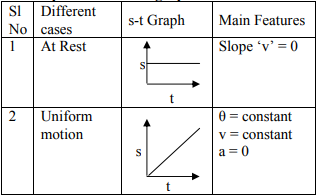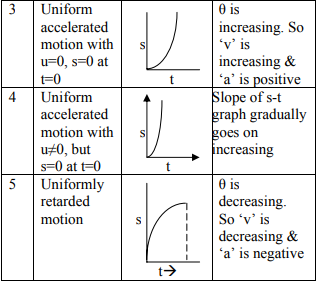Different cases of the velocity-time graph.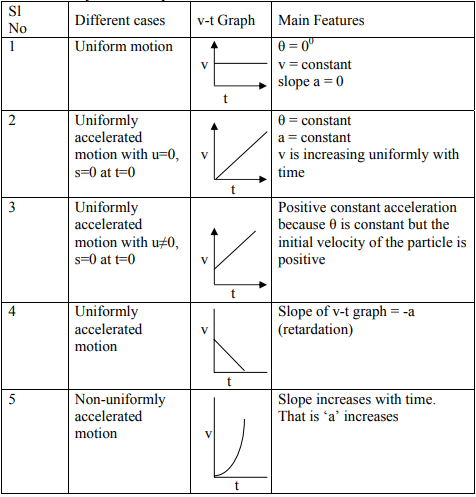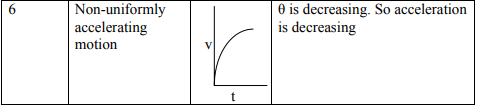## Types of Motion

### Projectile Motion

The motion of a body when it is thrown or projected with some initial velocity in a plane is known as projectile motion.

Examples:

• The motion of the golf ball.
• The motion of a rocket after burn-out
• The motion of a bomb dropped from an aeroplane etc.

Projectile motion is of two types;

1. Horizontal projection
2. Angular Projection

Horizontal Projection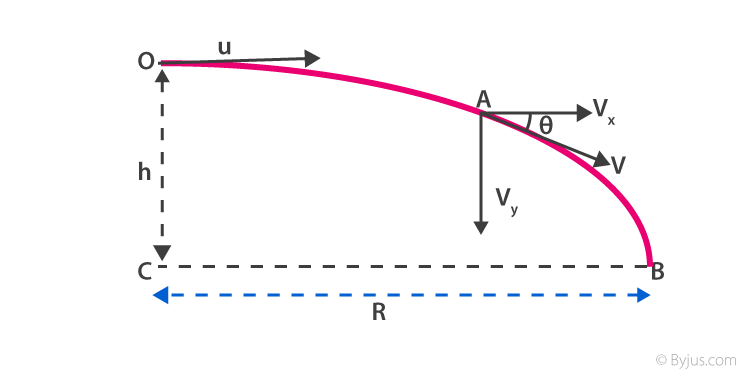When a body is projected along the horizontal direction with an initial velocity ‘u’ the projection is known as horizontal projection. It is assumed that there is negligible air resistance.

Velocity at any time t, v = √ [u2 + (gt)2]

The direction of velocity, θ = tan -1 (gt / u)

Time of flight, T = √2h / g

Range, R = u √2h / g

Angular Projection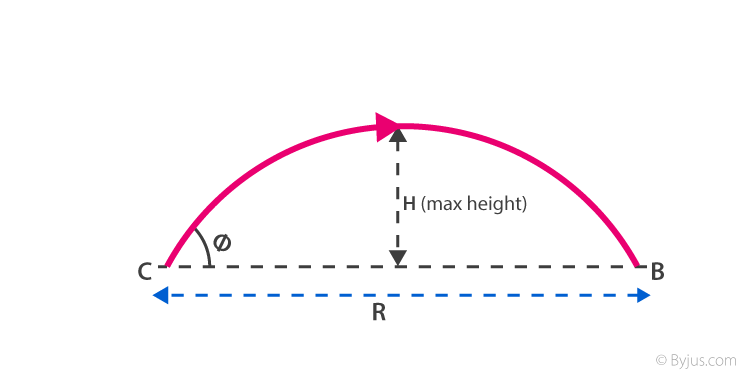When a body is thrown or projected at an angle with the horizontal direction is known as angular projection. It is assumed that air resistance is zero. The following equation of motion can be derived by examining the motion in the horizontal and vertical direction.

Equation of trajectory,

Y = x tan – ½ g [ x / u cos θ ] 2

Time of flight, T = (2u sin θ)/g

Maximum height, H = [u2 sin2 θ]/[2g]

Range, R = [u2 sin2 θ]/g

### Circular Motion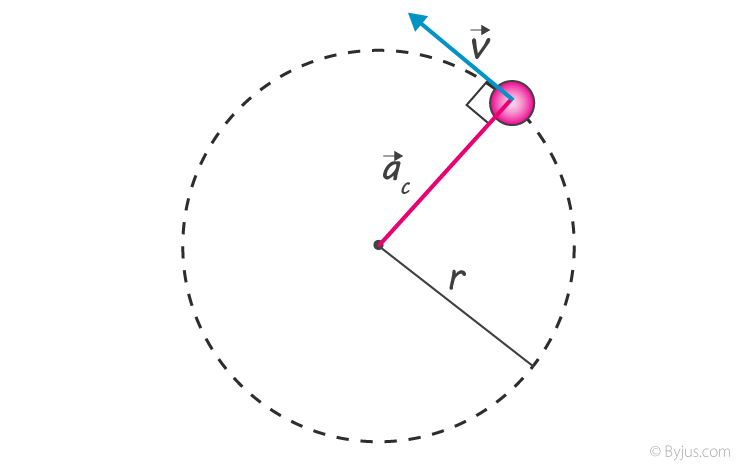The motion of an object along a circular path is called circular motion.

## Important Points to Remember

• When a body moves along a circular path at a constant speed, the motion of the body is known as uniform circular motion.
• The direction of velocity at any point in a circular motion is given by the tangent to the circle at that point.
• In a uniform circular motion, the velocity and acceleration both change due to continuous change in direction.
• When the speed of the keeps changing while moving along the circular path, the motion is called non-uniform circular motion.
• The magnitude of centripetal acceleration, ac = y2 / R